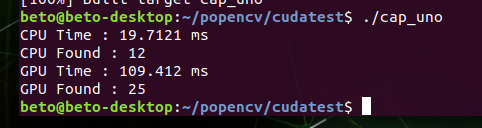# CUDA code too slow

Hi everybody, I’m newbie in Jetson nano & CUDA. I’m using Opencv 4.5 & Cuda 10.2, I’ve installed and compiled them succesufully in my nano board.
Just testing a code to learn about using CUDA and speed up the task, but I found using cuda I get low fps than without using it. Any ideas what am I doing wrong ? This is my test code:
cap_uno.cpp (3.3 KB)

Hi,

First, please make sure you have maximized the device performance.

``````\$ sudo nvpmodel -m 0
\$ sudo jetson_clocks
``````

This will help to figure out whether the performance issue is from GPU or data bandwidth.

Thanks.

Just running:

There is a bit of improvement in the results, but it’s still very slow using cuda filters, and it’s always faster without using cuda.
In short, I can’t find why my code using cuda, is slower than without it. What am I doing wrong ?

Still I’m stuck with this… :(
Now I’ve tried and opencv example that is in my “/usr/share/opencv4/samples/gpu” folder
That code is below: (I’ve just only change the name of the file, it is for test houghlines using cpu and gpu)
When I run that code I recieve:As you can see that’s my issue, gpu time is greather than cpu time.
Please, has someone an idea what is happening with my Jetson nano board ?
Thank you. Alberto.

``````#include <cmath>
#include <iostream>
#include "opencv2/core.hpp"
#include <opencv2/core/utility.hpp>
#include "opencv2/highgui.hpp"
#include "opencv2/imgproc.hpp"
#include "opencv2/cudaimgproc.hpp"

using namespace std;
using namespace cv;
using namespace cv::cuda;

static void help()
{
cout << "This program demonstrates line finding with the Hough transform." << endl;
cout << "Usage:" << endl;
cout << "./cap_uno <image_name>, Default is /data/pic1.png\n" << endl;
}

int main(int argc, const char* argv[])
{
const string filename = argc >= 2 ? argv : "data/pic1.png";

if (src.empty())
{
help();
cout << "can not open " << filename << endl;
return -1;
}

Mat dst_cpu;
Mat dst_gpu = dst_cpu.clone();

vector<Vec4i> lines_cpu;
{
const int64 start = getTickCount();

cv::HoughLinesP(mask, lines_cpu, 1, CV_PI / 180, 50, 60, 5);

const double timeSec = (getTickCount() - start) / getTickFrequency();
cout << "CPU Time : " << timeSec * 1000 << " ms" << endl;
cout << "CPU Found : " << lines_cpu.size() << endl;
}

for (size_t i = 0; i < lines_cpu.size(); ++i)
{
Vec4i l = lines_cpu[i];
line(dst_cpu, Point(l, l), Point(l, l), Scalar(0, 0, 255), 3, LINE_AA);
}

GpuMat d_lines;
{
const int64 start = getTickCount();

Ptr<cuda::HoughSegmentDetector> hough = cuda::createHoughSegmentDetector(1.0f, (float) (CV_PI / 180.0f), 50, 5);

hough->detect(d_src, d_lines);

const double timeSec = (getTickCount() - start) / getTickFrequency();
cout << "GPU Time : " << timeSec * 1000 << " ms" << endl;
cout << "GPU Found : " << d_lines.cols << endl;
}
vector<Vec4i> lines_gpu;
if (!d_lines.empty())
{
lines_gpu.resize(d_lines.cols);
Mat h_lines(1, d_lines.cols, CV_32SC4, &lines_gpu);
}

for (size_t i = 0; i < lines_gpu.size(); ++i)
{
Vec4i l = lines_gpu[i];
line(dst_gpu, Point(l, l), Point(l, l), Scalar(0, 0, 255), 3, LINE_AA);
}

imshow("source", src);
imshow("detected lines [CPU]", dst_cpu);
imshow("detected lines [GPU]", dst_gpu);
waitKey();

return 0;
}type or paste code here
``````

Hi,

Sorry for the late update.
Could you help to try the following experiments?

• Loop the process code to get the average execution time.

For example:

``````// CPU
const int64 start = getTickCount();
for(size_t i=0; i<iteraton; i++) {
cv::HoughLinesP(mask, lines_cpu, 1, CV_PI / 180, 50, 60, 5);
}
const double timeSec = (getTickCount() - start) / getTickFrequency() / iteration;

...

// GPU
Ptr<cuda::HoughSegmentDetector> hough = cuda::createHoughSegmentDetector(1.0f, (float) (CV_PI / 180.0f), 50, 5);
for(size_t i=0; i<warmup; i++) {
hough->detect(d_src, d_lines);
}

const int64 start = getTickCount();
for(size_t i=0; i<iteraton; i++) {
hough->detect(d_src, d_lines);
}
const double timeSec = (getTickCount() - start) / getTickFrequency() / iteration;
``````

Thanks.

Hi AastaLLL.
Yes obviously the times using cuda goes lower with your suggest. With values of warmup and iterations between 10-100 I get about the same results (cuda processing faster).
The point is: If I need to loop some functions when I use cuda, I waste a lot of time in iterations, warmup, instead using opencv functions that lost time does not exist.
So what is the advantage of using cuda functions ? Sorry I can’t deduct that,
Cheers.
Alberto.

This topic was automatically closed 14 days after the last reply. New replies are no longer allowed.